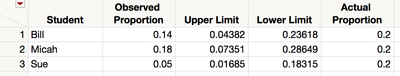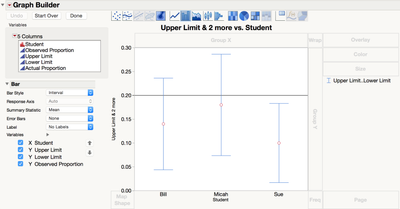Our World Statistics Day conversations have been a great reminder of how much statistics can inform our lives. Do you have an example of how statistics has made a difference in your life? Share your story with the Community!

## How to Display Multiple Confidence Intervals on One Plot

This posting is the result of a discussion with a high school statistics teacher.  The teacher has each student draw samples from a population, count the number of times a particular event occurs, and then construct a confidence interval for the proportion.  The true proportion is known.  The teacher wants to plot the confidence intervals for all of the students on one graph, and compare these intervals to the true proportion.

Here's one way to do this.

1.  Use the Confidence Interval for One Proportion calculator to construct confidence intervals for each student.  This can be found under Help > Calculators in JMP 12 SE, or under Help > Sample Data > Calculators in the full version of JMP.  Use the Summary Statistics option.

2.  Enter the confidence intervals into a data table structured like the table shown below.  This sample table is attached.3.  In Graph Builder (from the Graph menu), do the following (or run the Graph Builder script in the top left corner of the sample data table):

• Drag Student to the X-zone
• Drag the Upper Limit and Lower Limit to the Y zone (at the same time)
• Click on the Bar Chart icon (from the icons above the graph)
• On the left side, under Bar, change the bar style to Interval.
• Drag the Observed Proportion just inside the Y-axis until you see points appear within the intervals
• Double-click on the y-axis and in the bottom left corner add a reference line at 0.2 (you have to enter the value, and then click the Add button)If you're new to Graph Builder, the video below shows how to create this graph (there is no audio).

Article Labels
Article Tags
Contributors